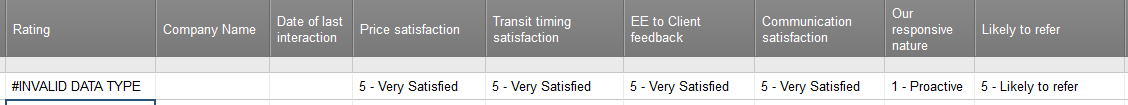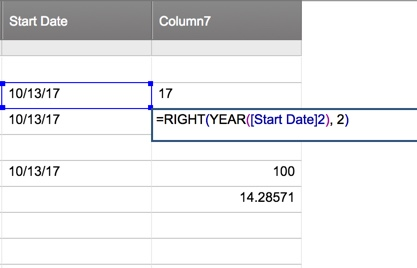#### Welcome to the Smartsheet Forum Archives

The posts in this forum are no longer monitored for accuracy and their content may no longer be current. If there's a discussion here that interests you and you'd like to find (or create) a more current version, please Visit the Current Forums.

# Extract a number from the Left of a cell - HELP!

Good Day to ALL.

I am new to the vommunity and need some help....

I have a survey form for my clients and when the results are populate, I would like to tally the results - by client.

What I am looking to do is extract the number to the left of the survey response.

eg. 5 - Very Satisfied

4 - Somewhat satisfied

3 - Neutral

I only want the numeric values - "5,4,3" etc. and have them SUM in a separate cell...

Any help is greatly appreciated.

Tags:

• You can use the following formula in a new column.

=SUM(VALUE(LEFT([Column1]4, 1)), VALUE(LEFT([Column2]4, 1)))

Use the brackets [   ] if your column name contains white space or numbers at the end. Basically, you are taking the leftmost character from the text value, and converting it to a number and summing them. Hope this helps.

• Mike,

Thanks for the help. I think I am almost there.

I have attached a screenshot of my sheet. I am needing to sum the numeric values of the 6 cells and populate the result in the Rating Column.

I hope the screen shot helps.

Thanks• Can you please post what you have as a formula in the Rating column?

• =SUM(VALUE(LEFT([Price satisfaction]7, 1)), VALUE(LEFT([Transit timing satisfaction]7, VALUE(LEFT([EE to Client feedback]7, VALUE(LEFT([Communication satisfaction]7, 1)))))))

• Try deleting all of the closing paragraphs at the end and posting it. Smartsheets will automatically close it for you.

• Thanks Mike. I tried the below:

=SUM(VALUE(LEFT([Price satisfaction], 1)), VALUE(LEFT([Transit timing satisfaction], VALUE(LEFT([EE to Client feedback], VALUE(LEFT([Communication satisfaction], 1)

and still get:

#UNPARSEABLE

Any Ideas?

• Okay, I think I see the issue. Each of the formulas nested in the SUM formula were not properly closed. And you were missing specific row numbers on two of them. Try this rendition!=SUM(VALUE(LEFT([Price satisfaction], 1)), VALUE(LEFT([Transit timing satisfaction]1)), VALUE(LEFT([EE to Client feedback]1)), VALUE(LEFT([Communication satisfaction], 1)))

• Thanks!!!

I used this minor edit and got it working.

Thank a million!

=SUM(VALUE(LEFT([Price satisfaction]1, 1)), VALUE(LEFT([Transit timing satisfaction]1, 1)), VALUE(LEFT([EE to Client feedback]1, 1)), VALUE(LEFT([Communication satisfaction]1, 1)), VALUE(LEFT([Our responsive nature]1, 1)), VALUE(LEFT([Likely to refer]1, 1)))

• How would you delete characters in a cell? I have a cell with the Year formula and want to drop the 20 from say 2018 and just leave the 18. Is there a way to that?

Bill

• Yes. =RIGHT(YEAR([Start Date]2), 2)

Replace my Year formula with your own...

See screenshot for how it's working.• This is an old post, so I'm hoping someone will see this question. I copied the working formula from the "deronbalch" response and SS keeps replacing the [ ] with ( ) and returning an error. Example: SS will 'convert' [Impact] to (Impact). What am I missing here?

=SUM(VALUE(LEFT([Total Expenditures]1, 2)),VALUE(LEFT([Impact]1, 2)), VALUE(LEFT([Resources]1, 2)), VALUE(LEFT([Duration]1, 2)), VALUE(LEFT([Innovation]1, 2)), VALUE(LEFT([Schedule Risk]1, 2), VALUE(LEFT([Budget Risk]1, 2), VALUE(LEFT([Quality Risk]1, 2), VALUE(LEFT([Data Sensitivity]1, 2)))

• You only need to add brackets around column names that have spaces or end in numbers. Remove the brackets from Impact, Duration and Innovation.

This discussion has been closed.